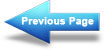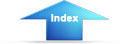Custom SearchChapter 2 - BatteriesMatter, Energy, and ElectricityConcepts of Alternating CurrentDIRECT CURRENT

LEARNING OBJECTIVES

Upon completing this chapter, you will be able to:

Identify the term schematic diagram and identify the components in a circuit from a simple schematic diagram. State the equation for Ohm's law and describe the effects on current caused by changes in a circuit. Given simple graphs of current versus power and voltage versus power, determine the value of circuit power for a given current and voltage. Identify the term power, and state three formulas for computing power.
Compute circuit and component power in series, parallel, and combination circuits. Compute the efficiency of an electrical device. Solve for unknown quantities of resistance, current, and voltage in a series circuit. Describe how voltage polarities are assigned to the voltage drops across resistors when Kirchhoff's voltage law is used. State the voltage at the reference point in a circuit. Define open and short circuits and describe their effects on a circuit. State the meaning of the term source resistance and describe its effect on a circuit. Describe in terms of circuit values the circuit condition needed for maximum power transfer. Compute efficiency of power transfer in a circuit. Solve for unknown quantities of resistance, current, and voltage in a parallel circuit. State the significance of the polarity assigned to a current when using Kirchhoff's current law. State the meaning of the term equivalent resistance. Compute resistance, current, voltage, and power in voltage dividers. Describe the method by which a single voltage divider can provide both positive and negative voltages. Recognize the safety precautions associated with the hazard of electrical shock. Identify the first aid procedures for a victim of electrical shock.INTRODUCTION

The material covered in this chapter contains many new terms that are explained as you progress through the material. The basic dc circuit is the easiest to understand, so the chapter begins with the basic circuit and from there works into the basic schematic diagram of that circuit. The schematic diagram is used in all your future work in electricity and electronics. It is very important that you become familiar with the symbols that are used.

This chapter also explains how to determine the total resistance, current, voltage, and power in a series, parallel, or combination circuit through the use of Ohm's and Kirchhoff's laws. The voltage divider network, series, parallel, and series-parallel practice problem circuits will be used for practical examples of what you have learned.Integrated Publishing, Inc. - A (SDVOSB) Service Disabled Veteran Owned Small Business